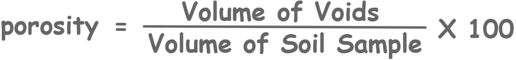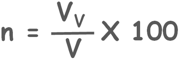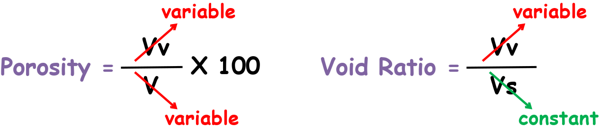PorosityA soil mass in general is composed of three kinds of matter,

1. Solids

2. Liquid

3. GaseousSolids can be minerals or organic matters or both. These solids can be of different sizes and shapes and because of it they enclose empty spaces. These empty spaces are called pores or voids.

The proportions of solids, water and air in a soil mass influence its physical properties. And before founding any structure on the soil we should study the soil in detail including its physical properties.

To determine these physical properties of soil we need to learn few simple terms which are frequently used in soil engineering.

Lets discuss one of these soil properties: porosity.

Porosity, which is denoted as small letter n, of a soil sample is defined as the ratio of the volume of voids that soil sample contains to the total volume of the sample, and is expressed as percentage.We can imagine a soil mass with its constituents (i.e. solids, water and air) separated, though these different constituents present in the soil mass cannot be separated, but it will be helpful in understanding the soil behavior and deriving its properties.

We can think of constituents occupying separate spaces. Volume of solids in the soil mass is marked as Vs, volume of water is as Vw and volume of air as Va.

Such diagrammatic representation of separated different phases of soil mass is called phase diagram.

From this phase diagram we can write porosity asNow as we know that volume of voids can never be zero in a soil sample, value of porosity will always be greater than zero

Volume of voids in any soil sample will never be greater than total volume of that sample because volume of voids is equal to the total volume of soil sample minus volume of solids present in the sample.

So even when volume of solids is equal to zero, volume of voids will be equal to the total volume, but never be greater than it.

Hence in the equation numerator will always be less than denominator hence the value of this ratio will always be less than 1.So we can Note here that the value of porosity can never be greater than or equal to 100%

Well technically we can say a water sample is a soil sample with volume of solids being equal to zero.

In soil engineering void ratio is more favoured for use than porosity. This is due to the fact that any change in the volume of soil mass is direct consequence of a similar change in the volume of voids while the volume of solids remains the same.Which means when soil mass changes its volume due to any external factors, there is change only its water content or its air content, which are in combined called volume of voids.

But these factors do not change the volume of solids.

In the equations of porosity and void ratiowe can see that on volume change porosity has two variables while void ratio has only one variable.

Tags : porosity

Published on :2019-08-04